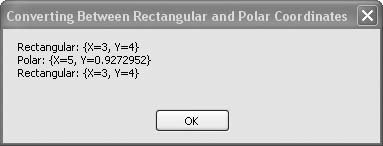# Recipe 6.13. Converting Between Rectangular and Polar Coordinates

#### Problem

You want to convert between two-dimensional coordinates expressed in either rectangular or polar notation.

#### Solution

Sample code folder: Chapter 06\ConvertPolar

Create two functions for the two conversions: ToPolar() and ToRectangular().

#### Discussion

The PointF structure provides a natural way to handle two-dimensional coordinates because each X, Y pair is handled as a single unit. A straightforward way to handle conversions between coordinates expressed in either rectangular (X, Y) or polar (radius, radians) notation is to simply pass and return PointF objects. This requires you, the programmer, to keep track of the current notation of each PointF object, but this is generally easy to do. Here are the two functions for making the conversions:

` Public Function ToPolar(ByVal sourcePoint As PointF) _       As PointF    ' ----- Convert   rectangular coordinates to polar.    Dim magnitude As Single    Dim radians As Single        magnitude = CSng(Math.Sqrt(sourcePoint.X ^ 2 + _       sourcePoint.Y ^ 2))    radians = CSng(Math.Atan2(sourcePoint.Y, sourcePoint.X))    Return New PointF(magnitude, radians) End Function Public Function ToRectangular(ByVal sourcePoint As PointF) _       As PointF    ' ----- Convert polar coordinates to rectangular.    Dim X As Single    Dim Y As Single    X = CSng(sourcePoint.X * Math.Cos(sourcePoint.Y))    Y = CSng(sourcePoint.X * Math.Sin(sourcePoint.Y))    Return New PointF(X, Y) End Function `

Both functions assume angles will be expressed in radians, which is consistent with the way angles are expressed in Visual Basic. You can convert angles to and from degrees using the constants presented in Recipe 6.10.

The following block of code demonstrates the use of the ToPolar() and ToRectangular() functions:

` Dim result As New System.Text.StringBuilder Dim pointA As PointF Dim pointB As PointF Dim pointC As PointF pointA = New PointF(3, 4) pointB = ToPolar(pointA) pointC = ToRectangular(pointB) result.AppendLine("Rectangular: " & pointA.ToString()) result.AppendLine("Polar: " & pointB.ToString()) result.AppendLine("Rectangular: " & pointC.ToString()) MsgBox(result.ToString()) `

The ToString() function presents the X and Y values of the PointF data using "X=" and "Y=" labels, which can be misleading when the PointF is holding a coordinate in polar mode. Be sure to keep track of the state of the data as you work with it.

Figure 6-13 shows the formatted string results of the ToRectangular() and ToPolar() functions in action.

##### Figure 6-13. Rectangular and polar two-dimensional coordinate conversions using PointF variablesVisual Basic 2005 Cookbook: Solutions for VB 2005 Programmers (Cookbooks (OReilly))
ISBN: 0596101775
EAN: 2147483647
Year: 2006
Pages: 400

Similar book on Amazon